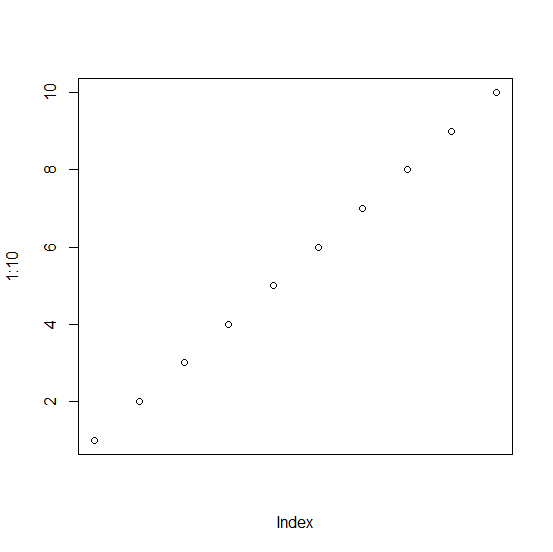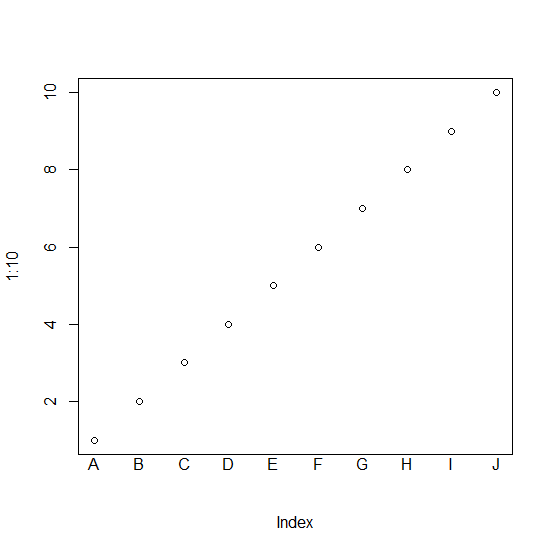# How to use mtext function to create the X-axis labels in base R?

The mtext function can help us to create X-axis or Y-axis labels and we can put these labels to places desired by us with the help of at argument. For example, if we want to use capital letters starting from A to J that are 10 characters on the X-axis labels then it can be done by using the below command −

mtext(text=LETTERS[1:10],outer=FALSE,side=1,las=1,at=1:10)

## Example

> plot(1:10,xaxt="n")

## Output## Example

> mtext(text=LETTERS[1:10],outer=FALSE,side=1,las=1,at=1:10)

## Output